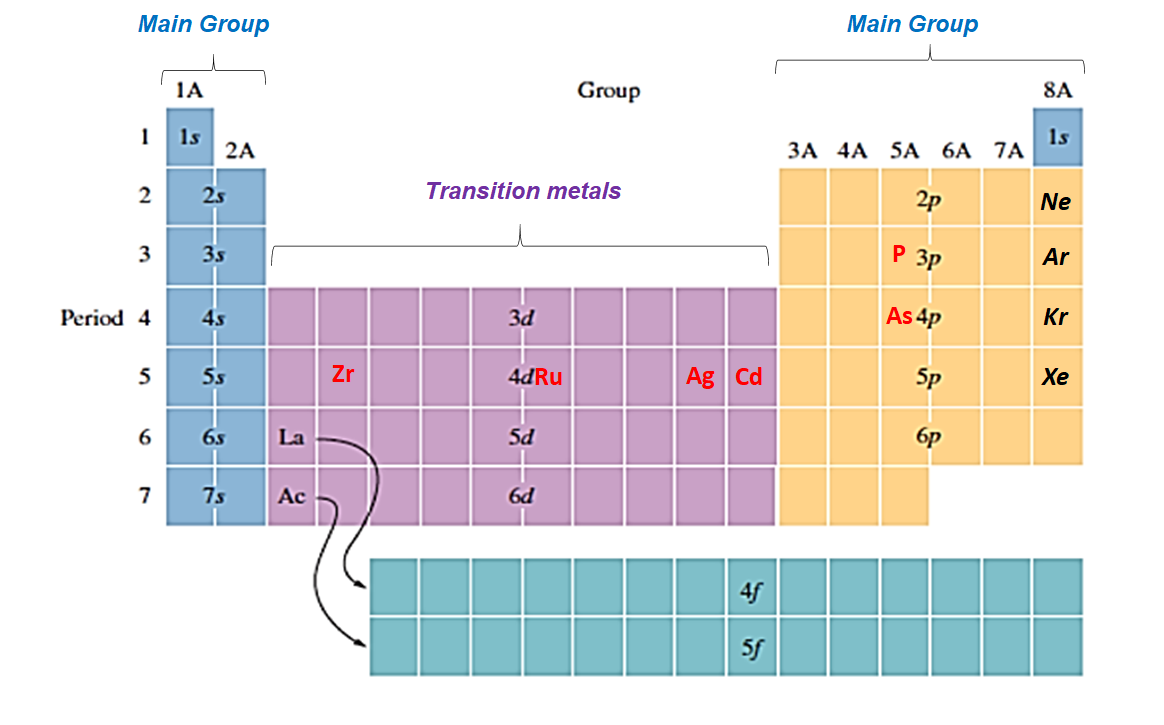# Problem: Write the electron configuration for the following ions, and determine which have noble-gas configurations:a. Cd2+b. P3- c. Zr4+ d. Ru3+e. As3-f. Ag+

###### FREE Expert Solution

We're asked to write the electron configuration for the following ions, and determine which have noble-gas configurations.

Recall that when an atom forms an ion it either gains or loses electrons:

• positive charge means valence electrons are removed or lost
• negative charge means electrons are added or gained

The valence electrons are found in the highest numbered orbital or higher energy orbital in a subshell.

• For main group(Group A) elements, the last or outermost orbital gains or loses the electron
• found in s, and p orbitals
• For transition metals (also known as Group B)the last s orbital loses an electron before the d orbitals.
• found in d orbital

For this problem, we need to do these steps:

Step 1: Determine # of electrons from atomic number using Periodic Table

Step 2: Write ground-state electron configuration

Ground-state means that the element is in its lowest energy form (not in excited state).

Step 3: Write electron configuration of each ion

Step 4:  Compare with nearest noble gas to determine whether it has a noble gas configuration

Recall that electron configurations are written from lower energy to higher energy orbital.

The following periodic table shows the orbitals in order of increasing energy levels: s < p < d < f:Recall that the distribution of electrons for each atomic orbital are:

s         _               1 orbital  can hold a maximum of 2 electrons

_ _ _            3 orbitals → can hold a maximum of 6 electrons

d       _ _ _ _ _       5 orbitals → can hold a maximum of 10 electrons

f      _ _ _ _ _ _ _   7 orbitals → can hold a maximum of 14 electrons

80% (66 ratings)###### Problem Details

Write the electron configuration for the following ions, and determine which have noble-gas configurations:

a. Cd2+
b. P3-
c. Zr4+
d. Ru3+
e. As3-
f. Ag+